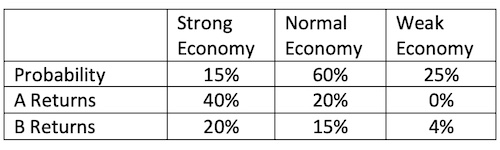### Why should I choose AnalystNotes?

Simply put: AnalystNotes offers the best value and the best product available to help you pass your exams.

##### Subject 8. Covariance Given a Joint Probability Function

We can calculate the covariance between two asset returns given the joint probability distribution. Consider the following example:

Suppose we wish to find the variance of each asset and the covariance between the returns of A and B, given that the amount invested in each company is \$1,000.

This table is used to calculate the expected returns:For us to find the covariance, we must calculate the expected return of each asset as well as their variances. The assets weights are: WA = 1000/2000 = 0.5 and WB = 1000/2000 = 0.5

Next, we should calculate the individual expected returns:
E(RA) = 0.15 x 0.40 + 0.60 x 0.2 + 0.25 x 0.00 = 0.18
E(RB) = 0.15 x 0.2 + 0.60 x 0.15 + 0.25 x 0.04 = 0.13

Finally, we can compute the covariance between the returns of the two assets:

Cov(RA, B) = 0.15 (0.40 - 0.18) (0.20 - 0.13) + 0.6 (0.20 - 0.18) (0.15 - 0.13) + 0.25 (0.00 - 0.18) (0.04 - 0.13) = 0.0066

Interpretation: Since covariance is positive, the two returns show some co-movement, though it's a weak one.

Learning Outcome Statements

l. calculate and interpret covariance given a joint probability function;

CFA® 2022 Level I Curriculum, Volume 1, Module 3

User Comment
anup5 Could somebody explain the detailed steps for the steps when we have a correlation matrix. eg : How to calculate variances ?
julescruis I dont understand the covariance matrix, anyone? (first graph way up)
epizi It is quite simple
Remember Cov(Ra,Ra)is same as Var(Ra)
Also Cov(Ra,Rb)=Cov(Rb,Ra)
So at the diagonals you will always find this common Cov(Ra,Ra),Cov(Rb,Rb) and Cov(Rc,Rc).
Note that you can always have this in mind able and estimate the number of covariance that can be found in the set of various variables.

Hope is clear now
epizi Sorry I explain something else,I think the first table is given, but if you must caculate the entries you may have to go back to the previous note just before this.
CraigSaba covariance matrix from example above - the covariance b/w A & B is 120. The variance of A is 625, of B is 196
challenge10 Anyone know how to derive the correlation of Rc with the portfolio Return of A & B in order to calculate the portfolio expected return of all 3 assets? Cheers as I'm wondering whether it would come up in the exam.
jorgeman81 As far as I understand, in this case the correlation matrix is given you can't calculate it with the given data.
Morginador How do you calculate the variance out of the correlation matrix?
ybavly I think jorgeman81 is correct. we need more information to calculate expected values and variance from the correlation matrix.
onyameba Can anybody help solve these questions?
1.If 100 random samples of size n were drawn and, if, for each, a 99% confidence interval for u was computed, then exactly 99 of these confidence intervals would contain the true population mean within their limits.
2.A dormitory on campus houses 200 students, 120 are male, 50 are upper division students, and 40 are upper division male students. A student is selected at random. Find the probability of selecting a lower division student, given the student is a female.
3. It is desired to test the claim that a steady diet of a medicine will cause a male to lose 10 lbs over 5 months. A random sample of 49 males was taken yielding an average weight loss over 5 months of 12.5 pounds, with s = 7 lbs.
a. Identify the critical value suitable for conducting a two-tail test of the hypothesis at the 2% level.
b. Is there any significant evidence that the pharmaceutical company claim about the drug performance is not valid, given an p=0.02? Show your work.
CJHughes Based on the notes given, I am a little confused how to back-out all of the last steps from the Correlation Matrix? If anyone could offer some assistance it would be greatly appreciated. Thanks
guest thank you epizi
ZainabA thank you epizi